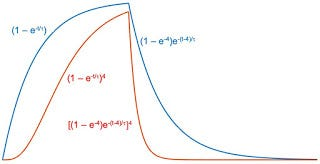# The Potassium Conductance

6.13.2 Potassium Conductance

Hodgkin and Huxley wanted a way to describe their extensive voltage-clamp data, similar to that in Figs. 6.34 and 6.35,. with a small number of parameters. If we ignore the small nonzero value of the conductance before the clamp is applied, the potassium conductance curve of Fig. 6.34 is reminiscent of exponential behavior, such as g_K(v,t) = g_K(v) (1 — e^(-t/tau(v))), with both g_K(v) and τ(v) depending on the value of the voltage. A simple exponential is not a good fit. Figure 6.36 shows why. The curve (1 — e^(-t/tau)) starts with a linear portion and is then concave downward. The potassium conductance in Figs. 6.34 and 6.35 is initially concave upward. The curve (1 — e^(-t/tau))^4 in Fig. 6.36 more nearly has the shape of the conductance data.

The experiment shows that whereas the potassium conductance rises with a marked delay it falls along an exponential type of curve which has no inflexion corresponding to that on the rising phase. [my italics]A plot of n(t) (blue) and n^4(t) (red) versus time. This is an extension of Fig. 6.36 in IPMB.

--

--